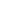Original research

# Comparing the efficiency of heart disease prediction using novel random forest, logistic regression and decision tree and SVM algorithms

## Abstract

Aim: The aim of the work is to evaluate the accuracy and precision in predicting heart disease using Support Vector Machine (SVM) , Random forest (RF), Logistic Regression (LR), Decision Tree (DT) Classification algorithms. Materials and Methods: Classification algorithm is appealed on a heart dataset which consists of 180 records. A framework for heart disease prediction in the medical sector comparing Random forest, Logistic Regression , Decision Tree and SVM classifiers has been proposed and developed. The sample size was calculated as 55 in each group using G power 80%. Sample size was calculated using clincalc analysis, with alpha and beta values 0.05 and 0.5, 95% confidence, pretest power 80% and enrolment ratio 1. Results: The Novel Random Forest Algorithm (92.13%) , Support Vector Machine (62.51%) , Logistic Regression (84.89%), Decision Tree (86.25%) classifiers produce respectively. SVM, RF exists a statistically significant difference between the two groups (p=0.001,p=.004;p<0.05).LR, RF exists a statistically insignificant difference between the two groups (p=.103, P=.080;p>0.05) both with confidence interval 95%. Hence Random forest is better than SVM, RF, DT classifiers. Conclusion: The results show that the performance of RF is better when compared with SVM, LR and DT in terms of both precision and accuracy.

## Imprint

P. Prasanna Sai Teja, Veeramani T. Comparing the Efficiency of Heart Disease Prediction using Novel Random Forest, Logistic Regression and Decision Tree And SVM Algorithms. Cardiometry; Issue 25; December 2022; p.1491-1499; DOI: 10.18137/cardiometry.2022.25.14911499; Available from: https://www.cardiometry.net/issues/no25-december-2022/comparing-efficiency-heart-disease

## Keywords

Logistic Regression,  Decision Tree,   Random Forest ,   Support Vector Machine,   Heart Disease Prediction,   Data MiningCardiometry in TelegramCurrent issueCardiometry's libraryFounders of Cardiometry